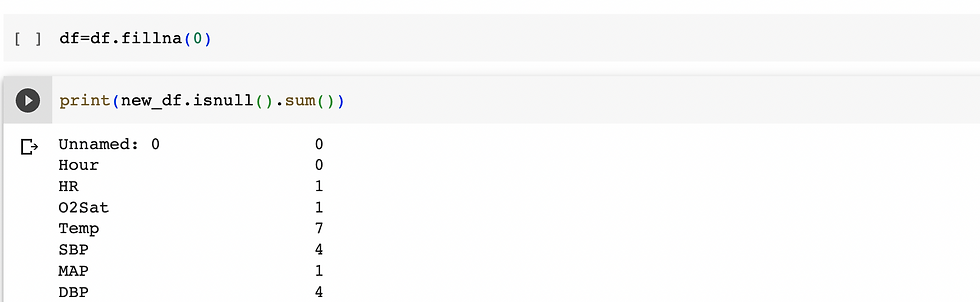top of pageSearch

# Exploratory Data Analysis

### Working on new datasets and trying to understand different variables in the data is a big challenge, but to understand the variables and the relationship between them exploratory data analysis comes handy. Exploratory data analysis is a best approach to understand the general patterns in the data and to find the data insights.

Below are the steps to follow in Exploratory data analysis :

1. Understanding the data : Head or tail of dataset, Shape of dataset, data types of column, describe dataset.

2. Data Cleaning : handling duplicates, missing values, handling null values, dropping unnecessary columns, feature selection.

3. Analyzing the the variables and the relationship between the variables.

Now in the below example sepsis dataset is used for exploratory data analysis :

1. Understanding the data :

After importing the libraries and the dataset we have to analyze the data.

```import pandas as pd
import numpy as np
import matplotlib.pyplot as plt
import seaborn as sns```
`df.head(5)``df.shape`
`df.info()``df.describe()`2. Data Cleaning :

After observing the data we can handle null values or missing values or outliers or deleting unnecessary columns etc.,

`df.columns.values`
`df.corr().head()`
`print(df.isnull().sum())`We can observe there are unwanted columns and null values in the given dataset, we can handle them in different ways.Now the unnecessary column is deleted.

Null values of one particular column can be filled or null values of all the data can also be filled.As we are handling clinical data instead of filling it with string like ‘Not Recorded’ or to fill random data we can fill with 0.Now we have cleaned the data with no null values.

3. Analyzing the the variables and the relationship between the variables. It can be done in three different ways:

1. Univariate Analysis : In univariate analysis we can analyze one variable only.

2. Bivariate Analysis : In Bivariate analysis we can analyze relationship between two variables.

3. Multivariate Analysis : In Multivariate analysis we can analyze relationship between more than two variables.

Univariate Analysis :

The analysis of no.of patients with and without sepsis is observed.The percentage of gender in given data is observed.Length of stay in ICU is analyzed using histogram.

```plt.figure(figsize=(10,6))
sns.distplot(df.ICULOS,color='r')
plt.title('Length of Stay in ICU',size=18)
plt.xlabel('',size=14)
plt.ylabel('',size=14)
plt.show()```Bivariate Analysis :

```#2.Bivariative :

plt.figure(figsize = (10,6))
sns.scatterplot(x='HR',y='DBP',color='r',data=df)
plt.title('HR vs DBP',size=18)
plt.xlabel('HR',size=14)
plt.ylabel('DBP',size=14)
plt.show()```The correlation between HR and DBP can also be observed as below :Pair plot of gender and age in data can also be observed.Multivariate Analysis:

We are analyzing the relation between Age, gender and sepsislabel in below example:We are analyzing the relation between gender, temperature and sepsislabel in below example:

```result = pd.pivot_table(data=df, index='Gender', columns='Temp',values='SepsisLabel')
print(result)
#create heat map of Gender vs Temp vs SepsisLabel
sns.heatmap(result, annot=True, cmap = 'RdYlGn', center=0.117)
plt.show()```After performing Exploratory Data Analysis(EDA) on the dataset , basic understanding of data , data cleaning and exploring the data using graphs can also be observed.